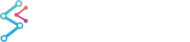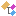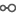﻿ SciChart.Charting.Visuals.Axes.LogarithmicAxis Namespace | WPF Chart DocumentationSciChart.Charting Assembly : SciChart.Charting.Visuals.Axes.LogarithmicAxis Namespace
SciChart.Charting.Visuals.Axes.LogarithmicAxis Namespace
Classes
ClassDescriptionProvides a Logarithmic, Value Numeric Axis, capable of rendering double, int, short, byte, long ticks on the X or Y-Axis of a SciChart.Charting.Visuals.SciChartSurface. The LogarithmicBase property determines which base is used for the logarithm.Provides a Logarithmic, Value Numeric Axis, capable of rendering negative double, int, short, byte, long ticks on the X or Y-Axis of a SciChart.Charting.Visuals.SciChartSurface. The LogarithmicBase property determines which base is used for the logarithmic scale.
Interfaces
InterfaceDescriptionDefines the interface to a logarithmic axis, the value axis which uses a logarithmic scale. The LogarithmicBase property determines which base is used for the logarithm.